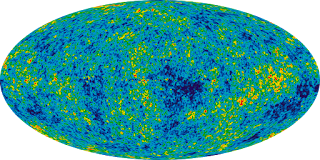## Thursday, November 28, 2019

### The problem with the Hubble constantCosmic Microwave Background RadiationNASA-WMAP

The Hubble constant, which measures the speed of expansion of space in the universe, has very curious properties. For instance, although we call it constant, it turns out that it is not a constant, as it varies over time. That is why its current value is represented by the symbol H0, but since its value was different at other times, it can be represented by other symbols, such as HCMBR, which refers to its value at the time when the cosmic microwave background radiation originated, about 13.7 billion years ago.

One of the hot issues of research is the current value of the Hubble constant. The way to calculate it seems quite simple: it consists in dividing the speed of expansion of space in our vicinity by the length of that space. To do this, the classic way to do it is to measure the distance of a relatively close galaxy (a few hundred light-years), and measure the speed with which it moves away from us. The second value is divided by the first, and we have the approximate value of H0.
But the analysis of the cosmic background radiation thanks to the COBE, WMAP and Planck satellites provided another independent way to calculate the Hubble constant, which consists in proposing a standard cosmological model, adjusting it to the data provided by the satellites about the acoustic oscillations in said radiation, and deduce from there the value of H0. In this case its value is not measured directly, but the adjusted standard model applies Einstein’s cosmological equations to calculate it.Edwin Powell Hubble
The problem is that the two results obtained do not tally. When variable Cepheid stars or supernovae are used to estimate the distance of a galaxy, and the redshift of the same galaxy to estimate the speed with which it moves away from us (i.e. the speed of expansion of space), we get a value around 74 with an accuracy of plus or minus 1.4 km/sec/Megaparsec. On the other hand, when it is calculated using the standard cosmological model, the value that comes out is 67.4 plus or minus 0.5 km/sec/Mpc.
At first glance it may seem a small difference, but it is almost 10%, while both independent results claim to achieve much greater accuracy. The situation for cosmological physics is unsustainable. To explain the discrepancy, two alternatives have been proposed:
1. The distance measurements of nearby galaxies are wrong. We must find new methods to perform them, until both calculations fit.
2. The standard cosmological model (called ΛCDM model) is wrong. We must give it up and design another model.
Several new methods have lately been proposed to calculate the distance of nearby galaxies with greater precision. Among them we can mention the following:
• Instead of variable cepheids or supernovae, red giants have been used to estimate the distance. The result was 69.8, halfway between the two conflicting results.
• Gravitational lenses can also be used, when a galaxy interposes between us and a distant quasar, whose light curves as it passes near the galaxy, giving rise to a multiple image, from which the distance of the galaxy can be deduced. This method has resulted in H0 = 73.3, very close to that obtained by traditional methods.
• Another team has calculated the distance from megamasers, gas clouds revolving around a black hole and emitting light of a certain wavelength. The result obtained was again equal to 74.
• Another method based on image pixelation resulted in the value 76.5.
• A value of 73.6 was obtained from the use of certain special stars (Miras) instead of cepheids.
• Finally, a new method based on the analysis of recently discovered gravitational waves has given the intermediate result of 70, although so far only a single experiment has been possible, so the accuracy is much lower than with the others methods (plus or minus 10).
In conclusion: most of the methods based on the direct measurement of the current value of the Hubble constant are grouped around the value 74. The standard cosmological model gives a much lower value: 67.4. Two of the new methods give intermediate values, close to 70, although one of them (gravitational waves) cannot yet be considered accurate.
This discrepancy, which can be seen graphically in a figure in this Science News article that shows simultaneously the different results and their accuracy, has led many astronomers to consider whether the time has come to review the standard cosmological model, which also presents other problems, like those I mentioned in another post in this blog.

The same post in Spanish
Manuel Alfonseca